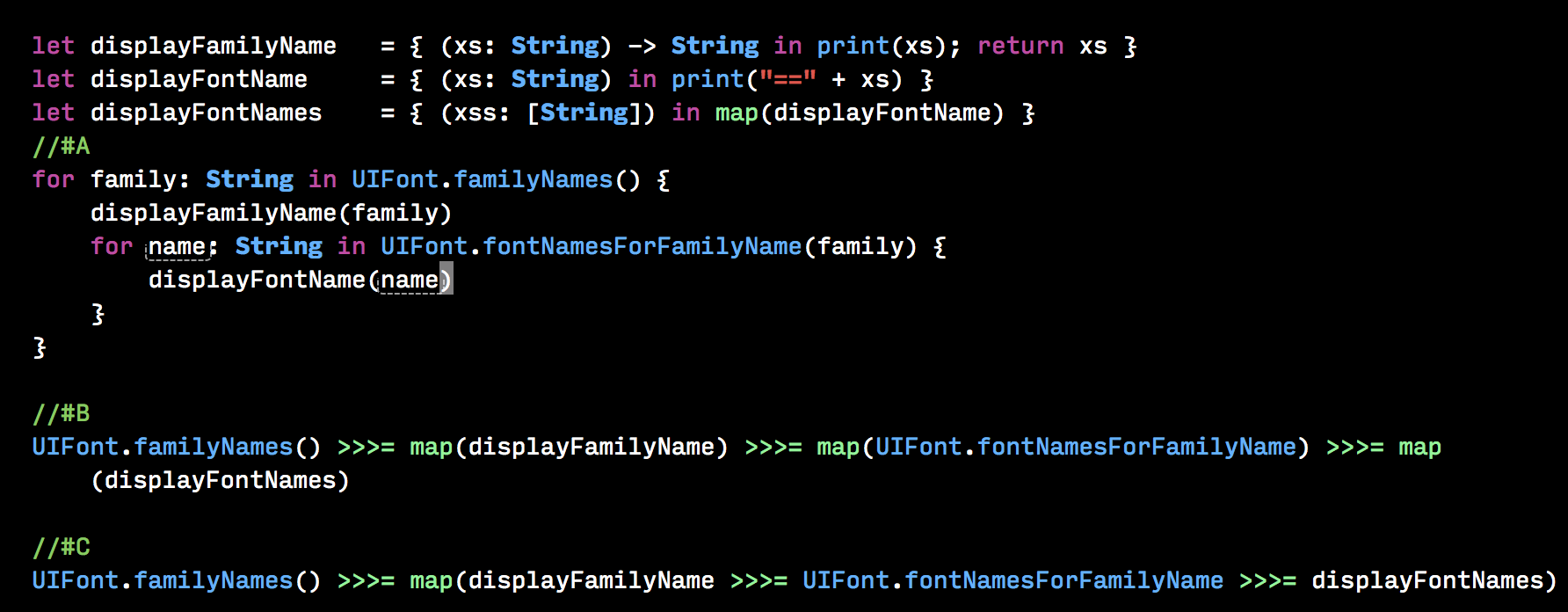### 1.0.6

A functional library for Swift programmers

### 1.0.6

###### 2019-11-13T15:57:56Z

A functional library for Swift programmers.

It contains a collection of useful functional libraries.

### Data

Data.Char, Data.List, Data.Maybe, Data.Either, Data.Tuple, Data.Function

Data.Num

Prelude

# Prerequisites

macOS 10.13 Xcode 9+

# Examples

## Data.List

### map

``````let xs = "haskell"
map(toUpper, xs)
``````

`"HASKELL"`

### filter

``````let xs               = [1, 2, 3, 4, 5]
let greaterThanThree = { x in x > 3 }
filter(greaterThanThree, list)
``````

`[4, 5]`

### foldl

``````let xs       = [1, 2, 3]
let adds     = { (x: Int,y: Int) in x+y }
``````

`6`

## Data.Function

### .. (Function Composition)

#### 1

``````let process : ([Int]) -> Int = last .. reverse
let xs       = [1,2,3,4,5]
process(xs)
``````

`1`

#### 2

``````func _isPrime(n : Int) -> Bool {
let array2ToN : Int->[Int]   = { x in Array(2...x) }
let divisors  : Int->[Int]   = array2ToN .. Int.init .. ceil .. sqrt .. Double.init
let isDivisible              = or .. map({ x in n % x == 0})
let isNotPrime               = isDivisible .. divisors
return !isNotPrime(n)
}
_isPrime(3)
``````

`true`

## Data.Maybe

### maybeToList

``````let x : Int? = nil
maybeToList(x)
``````

`[]`

### catMaybes

``````let xs: [Int?] = [1, nil, 3]
catMaybes(xs)
``````

`[1,3]`

### How does it help?

There are 3 solutions to the same problem. A, B, CA doesn't use HaskellSwift, B and C use HaskellSwift. A is imperative, B and C are declarative.

If you want to know more about it, please check the test cases in the Xcode Project or use Hoogle.